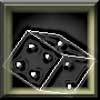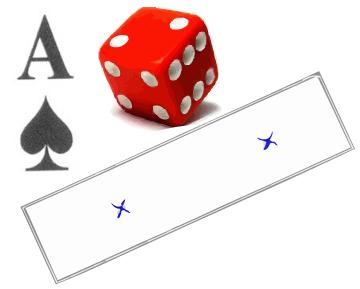#### You may also like### Gambling at Monte Carlo

A man went to Monte Carlo to try and make his fortune. Is his strategy a winning one?### Marbles and Bags

Two bags contain different numbers of red and blue marbles. A marble is removed from one of the bags. The marble is blue. What is the probability that it was removed from bag A?### Coin Tossing Games

You and I play a game involving successive throws of a fair coin. Suppose I pick HH and you pick TH. The coin is thrown repeatedly until we see either two heads in a row (I win) or a tail followed by a head (you win). What is the probability that you win?

# Distribution Differences

##### Age 14 to 16Challenge LevelWhen we collect and compare data for a subject or question that interests us we often consider and compare the mean or median of each data set. We might also try to measure how dispersed the data is in each case, using the standard deviation or the inter-quartile range, and perhaps using a box plot as a convenient display.

When we compare situations which include a value that varies randomly we need to do something similar.

But there's a difference: any data with a random variable are only a sample, take the data again and they will almost certainly be different. For example, if you throw a die 100 times and draw a graph this will almost certainly be different from the next time you throw it 100 times.

On the other hand, if you take the height measurements for a group of people, you don't expect the measurements to be different if you immediately measure them again.

In this problem you'll use your critical thinking skills to consider how best to compare probability distributions. Several of the questions posed below are there to start you thinking.

The main questions for you to answer are :

• How is Aces High like Five Dice ? How is it different ? And what if this involved tossing a coin rather than dice and cards ?

• 10cm is different to Five Dice in some key ways - what would you say those differences were ?Five Dice - When five dice are rolled together which do you expect to see more often, no sixes or all sixes?

What is the probability for no sixes, or for one six only, or two sixes, three, four, or all five?

10cm - A group of people are asked to put two marks on a piece of paper an estimated 10cm apart, what fraction of the group do you think will be within half a centimetre of the target length?

Aces High - One hundred people have each shuffled a pack of cards. They turn the cards one by one keeping in time with each other. If they get an Ace of Spades they hold it up. An observer records how many aces were held up at each of the synchronised card turns as each member of the group works their way through their shuffled pack. How many of those 52 observations do you think were zero? How about one, or two, ...?

Now each of those three situations has a probability distribution associated with it.

In Five Dice the number of sixes could be zero, or five, or something in between. Each of those events has a likelihood of happening, and because one of them must happen the sum of all the probabilities together is 1. Those probabilities considered together are called the 'probability distribution'

In the activity estimating 10cm the results will probably spread below and above the target length. The 'probability distribution' for that spread would describe how likely a person chosen at random would be to be within a certain distance of the target length. There would probably be more chance of being within one centimetre of 10cm than of being off by between 5cm and 6cm. Do you think estimating too much is as likely as too little ? You would need an experiment to inform your view on that.
• How is Aces High like Five Dice ? How is it different? And what if this involved tossing a coin rather than dice and cards?

• 10cm is different to Five Dice in some key ways - what would you say those differences were?